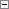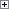Represents the difference between two DateTimes. This value can be negative. It is stored as a 64-bit integer. The integer value is the number of milliseconds during the duration.

The following are examples of durations:

DATETIME-DATETIME=DURATION

DATETIME-DURATION=DATETIME

DATETIME+DURATION=DATETIMEExample

This example shows how to calculate the difference between two DateTimes. It requires that you define the following variables.

Variable DataType

DateTime1

DateTime

Datetime2

DateTime

Duration

Duration

This example is run on a computer with the Current Format in the Regional and Language Options set to English (United States).Copy Code
DateTime1 := CREATEDATETIME(010109D, 080000T); // January 1, 2009 at 08:00:00 AM
DateTime2 := CREATEDATETIME(050509D, 133001T); // May 5, 2009 at 1:30:01 PM
Duration := DateTime2 - DateTime1;
MESSAGE(FORMAT(Duration));

The message window displays the following:

124 days 4 hours 30 minutes 1 secondSee Also

Reference

DateTime Data Type